Скачать презентацию Three universal oscillatory asymptotic phenomena Michael Berry Physics

97ca89efecb41c1d8b7a3d234f6180f2.ppt

• Количество слайдов: 37Three universal oscillatory asymptotic phenomena Michael Berry Physics Department University of Bristol United Kingdom http: //www. phy. bris. ac. uk/staff/berry_mv. html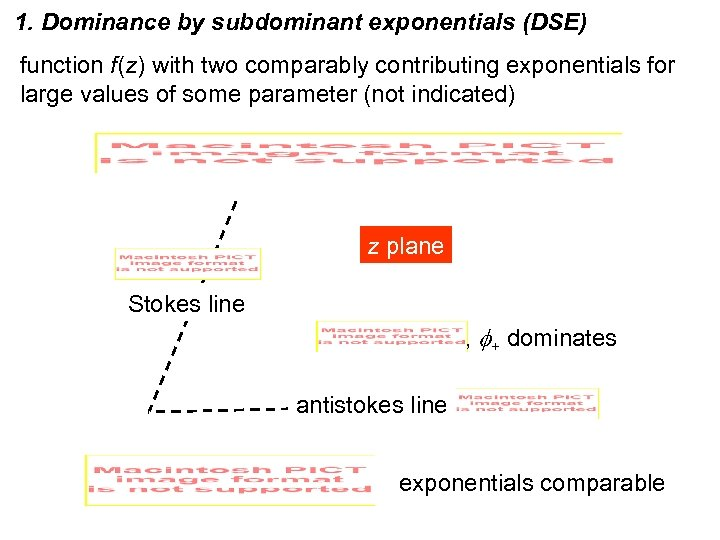1. Dominance by subdominant exponentials (DSE) function f(z) with two comparably contributing exponentials for large values of some parameter (not indicated) z plane Stokes line , f+ dominates antistokes line exponentials comparable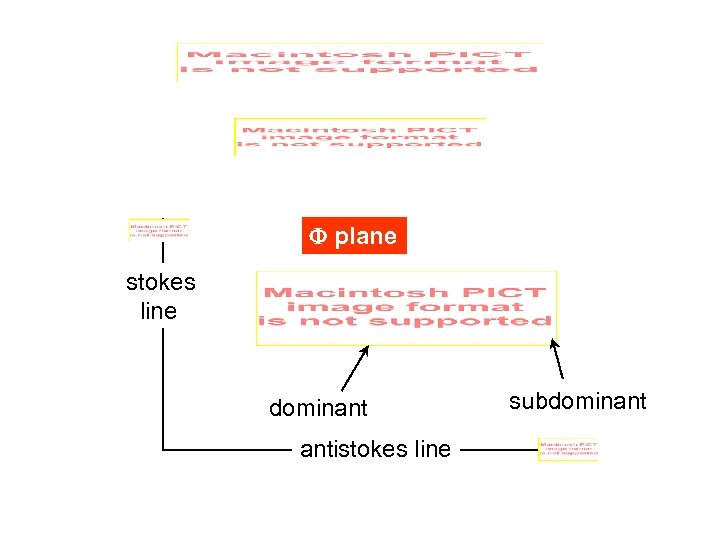F plane stokes line dominant antistokes line subdominant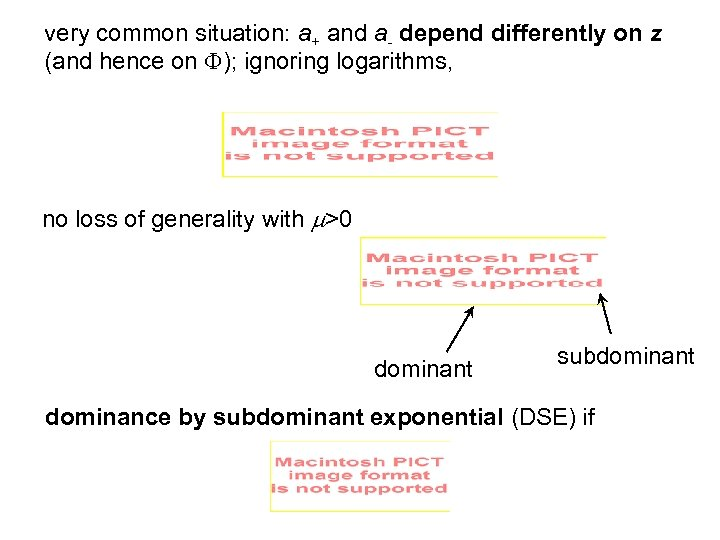very common situation: a+ and a- depend differently on z (and hence on F); ignoring logarithms, no loss of generality with m>0 dominant subdominant dominance by subdominant exponential (DSE) if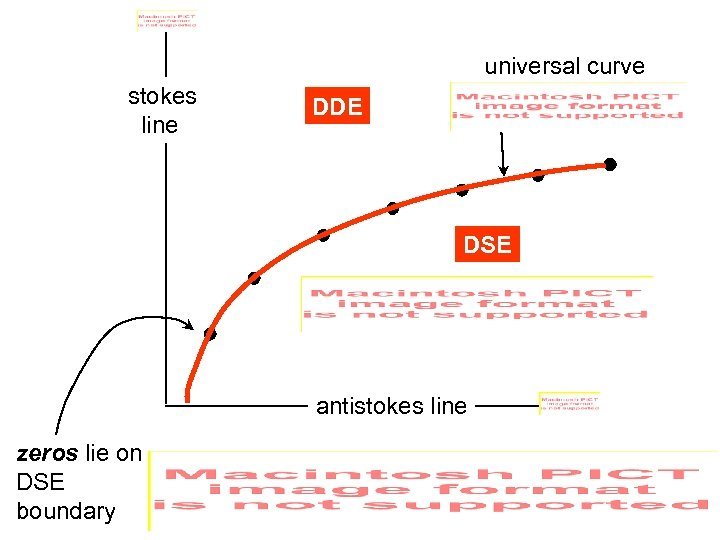universal curve stokes line DDE DSE antistokes line zeros lie on DSE boundary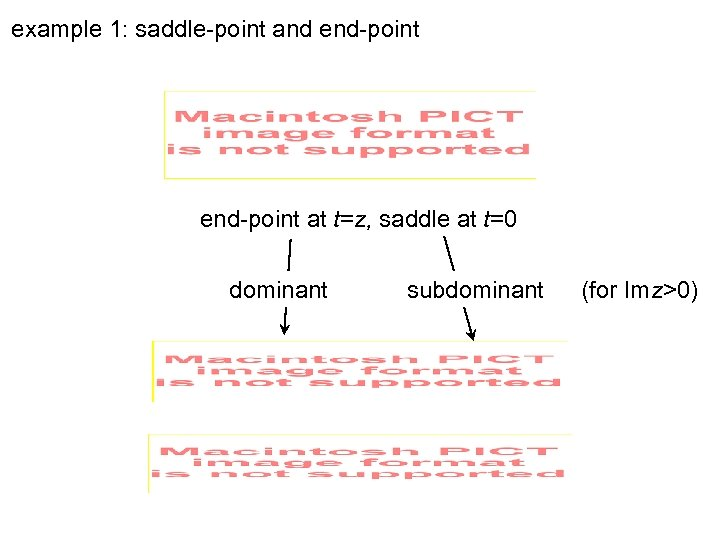example 1: saddle-point and end-point at t=z, saddle at t=0 dominant subdominant (for Imz>0)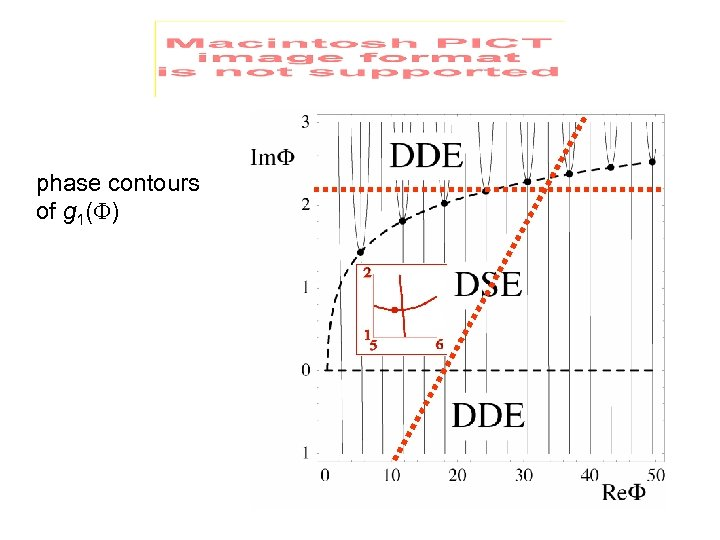phase contours of g 1(F)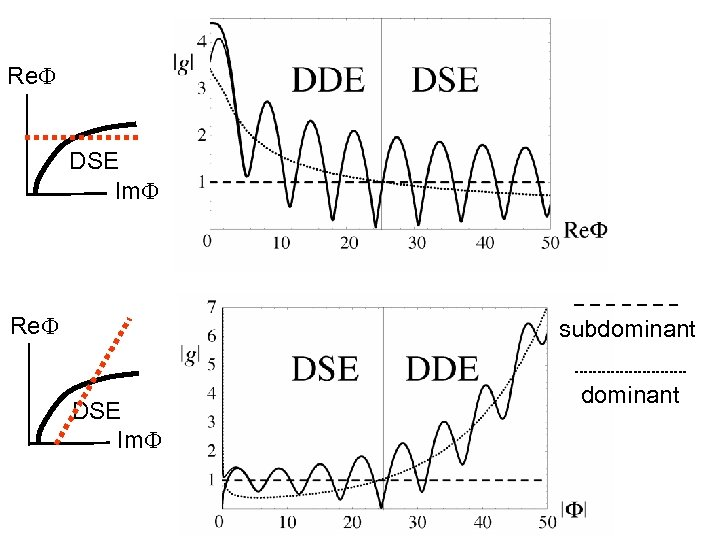Re. F DSE Im. F Re. F subdominant DSE Im. F dominant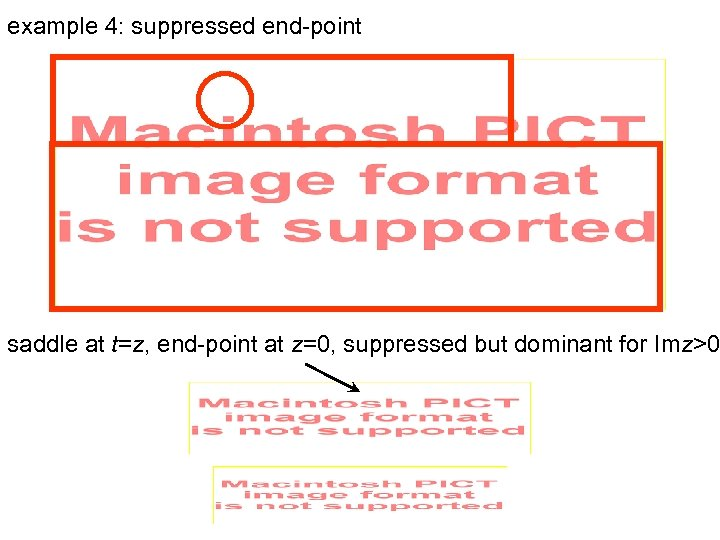example 4: suppressed end-point saddle at t=z, end-point at z=0, suppressed but dominant for Imz>0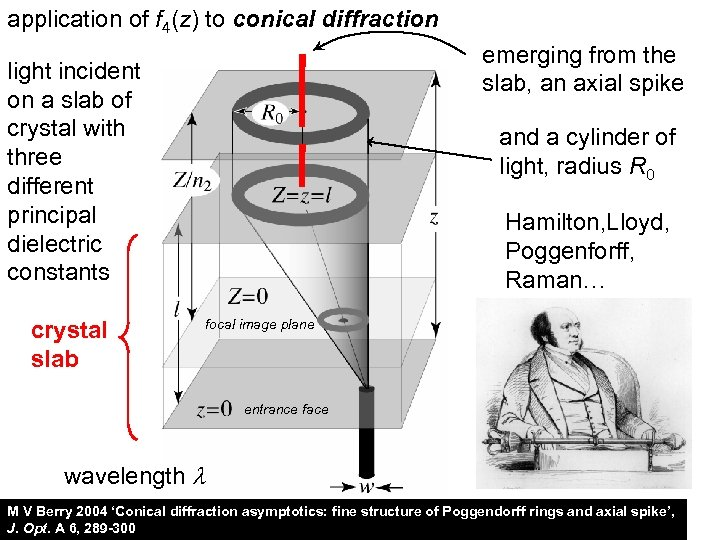application of f 4(z) to conical diffraction emerging from the slab, an axial spike light incident on a slab of crystal with three different principal dielectric constants crystal slab and a cylinder of light, radius R 0 Hamilton, Lloyd, Poggenforff, Raman… focal image plane entrance face wavelength l M V Berry 2004 ‘Conical diffraction asymptotics: fine structure of Poggendorff rings and axial spike’, J. Opt. A 6, 289 -300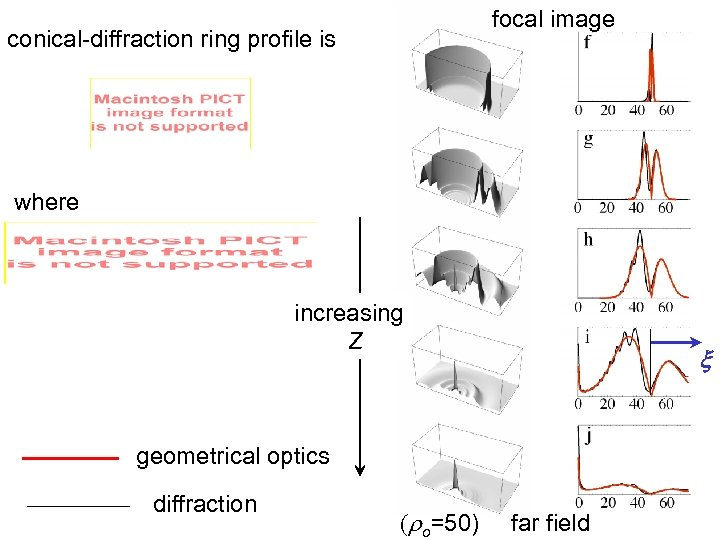focal image conical-diffraction ring profile is where increasing Z x geometrical optics diffraction (ro=50) far field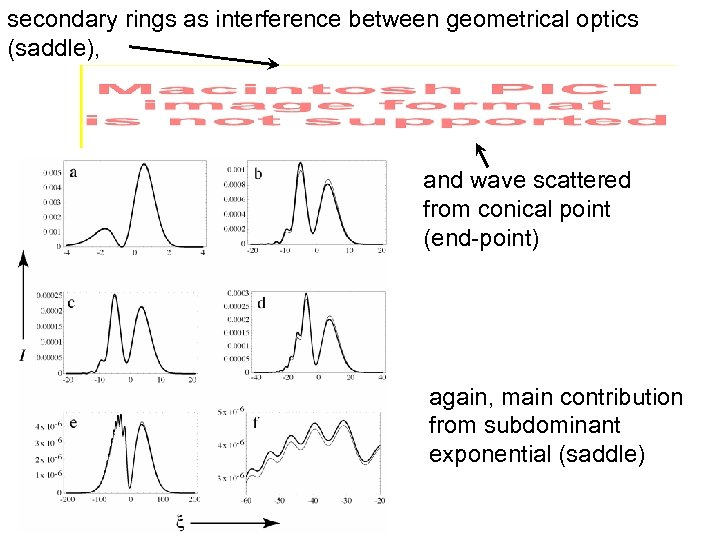secondary rings as interference between geometrical optics (saddle), and wave scattered from conical point (end-point) again, main contribution from subdominant exponential (saddle)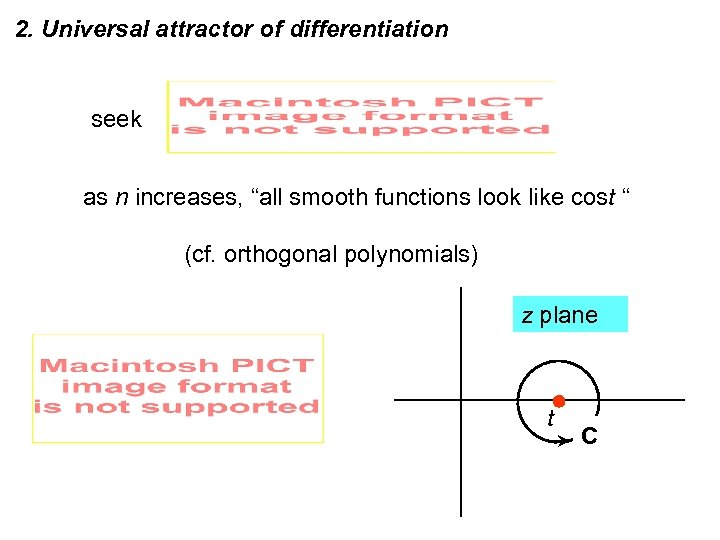2. Universal attractor of differentiation seek as n increases, “all smooth functions look like cost “ (cf. orthogonal polynomials) z plane t C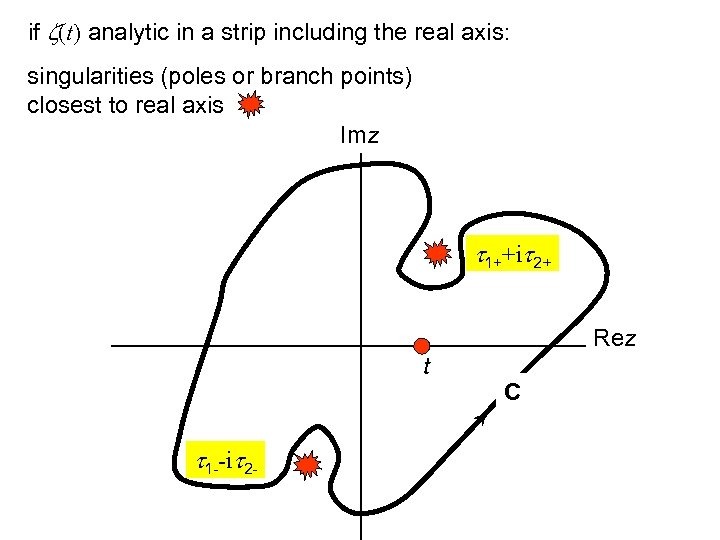if z(t) analytic in a strip including the real axis: singularities (poles or branch points) closest to real axis Imz t 1++it 2+ Rez t t 1 --it 2 - C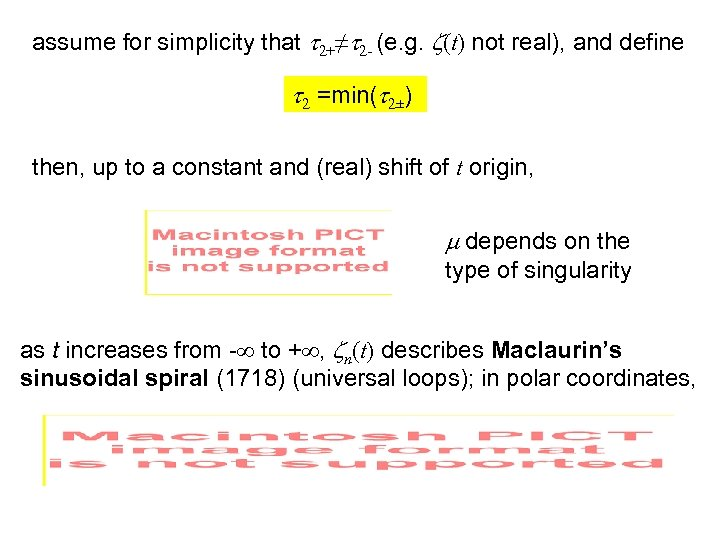assume for simplicity that t 2+≠t 2 - (e. g. z(t) not real), and define t 2 =min(t 2±) then, up to a constant and (real) shift of t origin, m depends on the type of singularity as t increases from - to + , zn(t) describes Maclaurin’s sinusoidal spiral (1718) (universal loops); in polar coordinates,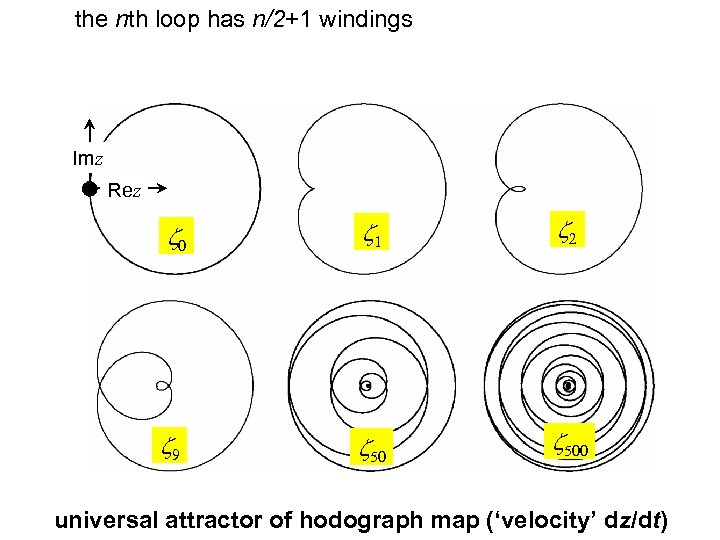the nth loop has n/2+1 windings Imz Rez z 0 z 1 z 2 z 9 z 500 universal attractor of hodograph map (‘velocity’ dz/dt)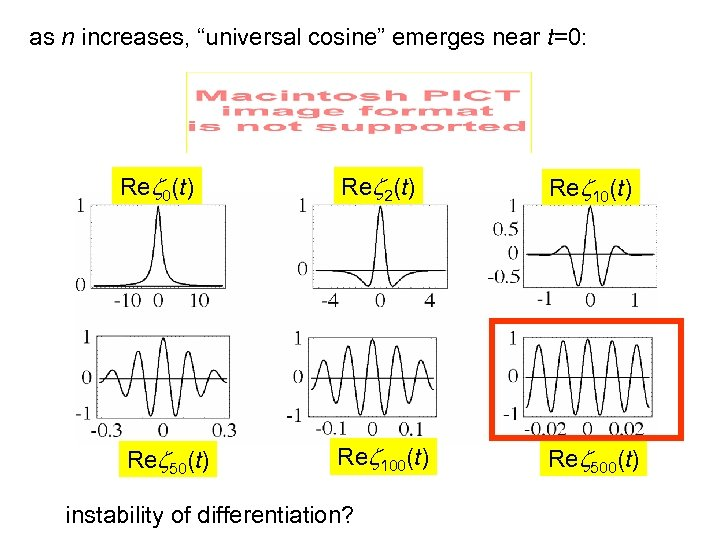as n increases, “universal cosine” emerges near t=0: Rez 0(t) Rez 2(t) Rez 10(t) Rez 50(t) Rez 100(t) Rez 500(t) instability of differentiation?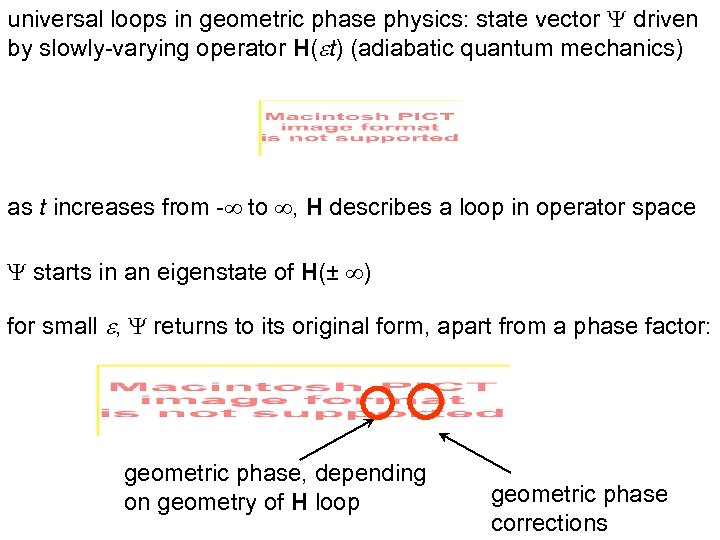universal loops in geometric phase physics: state vector Y driven by slowly-varying operator H( t) (adiabatic quantum mechanics) as t increases from - to , H describes a loop in operator space Y starts in an eigenstate of H(± ) for small , Y returns to its original form, apart from a phase factor: geometric phase, depending on geometry of H loop geometric phase corrections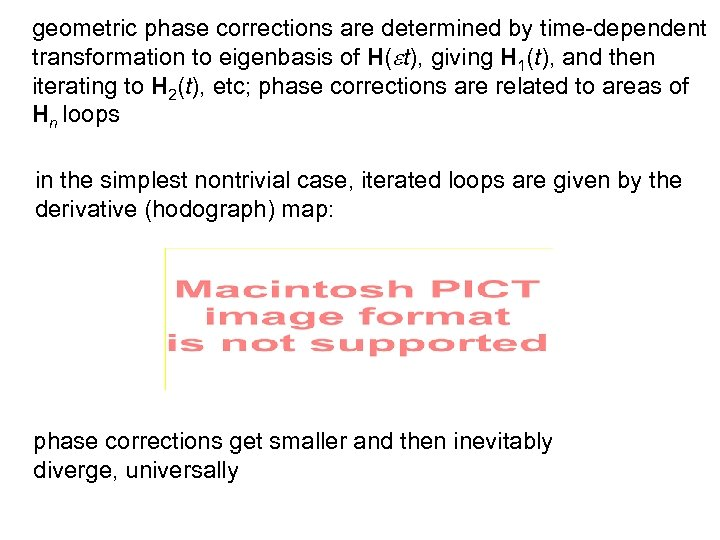geometric phase corrections are determined by time-dependent transformation to eigenbasis of H( t), giving H 1(t), and then iterating to H 2(t), etc; phase corrections are related to areas of Hn loops in the simplest nontrivial case, iterated loops are given by the derivative (hodograph) map: phase corrections get smaller and then inevitably diverge, universally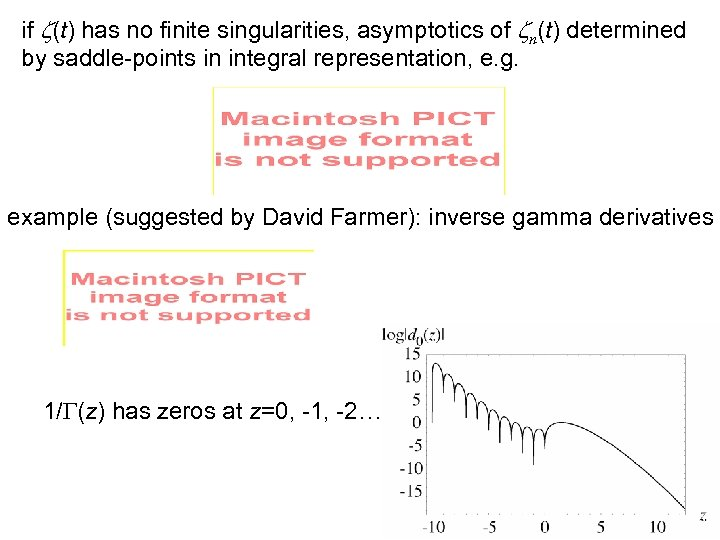if z(t) has no finite singularities, asymptotics of zn(t) determined by saddle-points in integral representation, e. g. example (suggested by David Farmer): inverse gamma derivatives 1/G(z) has zeros at z=0, -1, -2…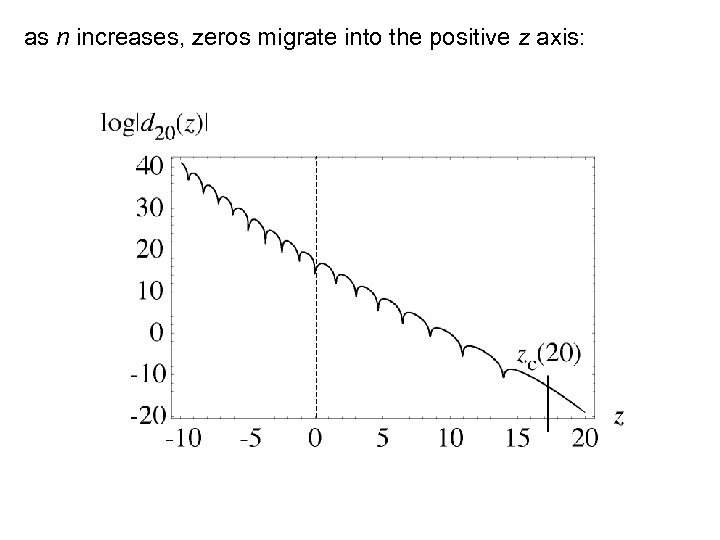as n increases, zeros migrate into the positive z axis: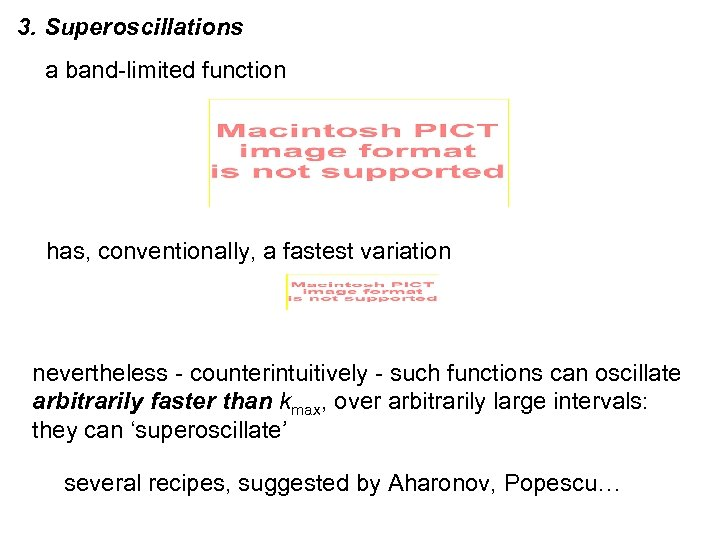3. Superoscillations a band-limited function has, conventionally, a fastest variation nevertheless - counterintuitively - such functions can oscillate arbitrarily faster than kmax, over arbitrarily large intervals: they can ‘superoscillate’ several recipes, suggested by Aharonov, Popescu…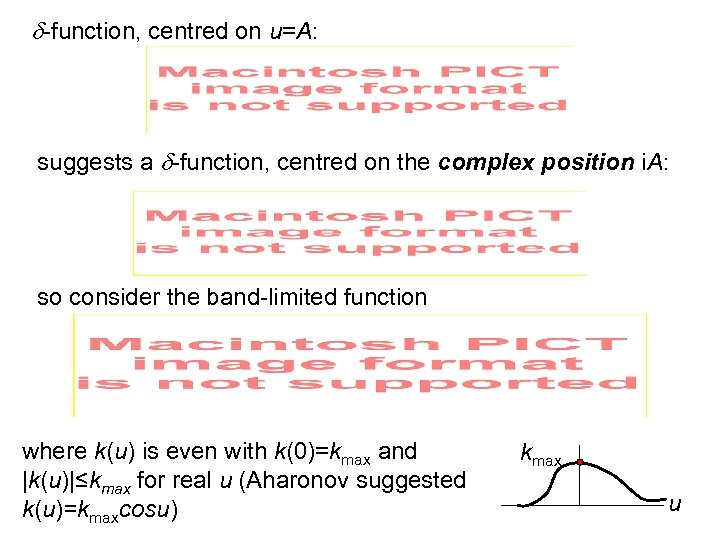-function, centred on u=A: suggests a -function, centred on the complex position i. A: so consider the band-limited function where k(u) is even with k(0)=kmax and |k(u)|≤kmax for real u (Aharonov suggested k(u)=kmaxcosu) kmax u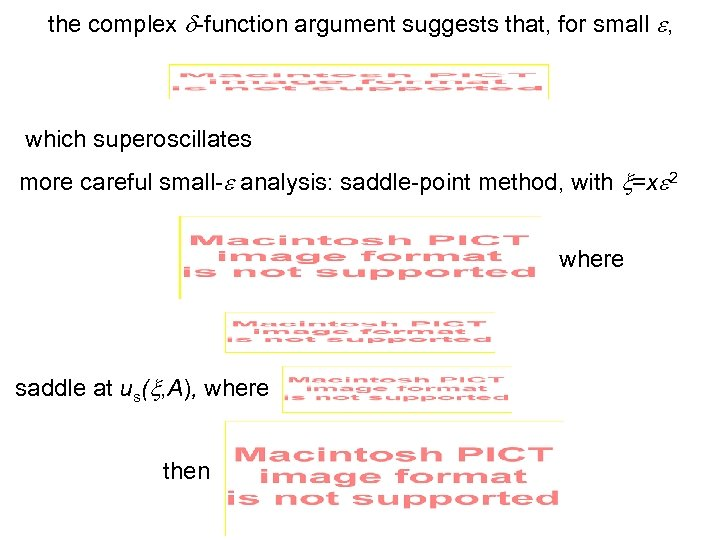the complex -function argument suggests that, for small , which superoscillates more careful small- analysis: saddle-point method, with =x 2 where saddle at us( , A), where then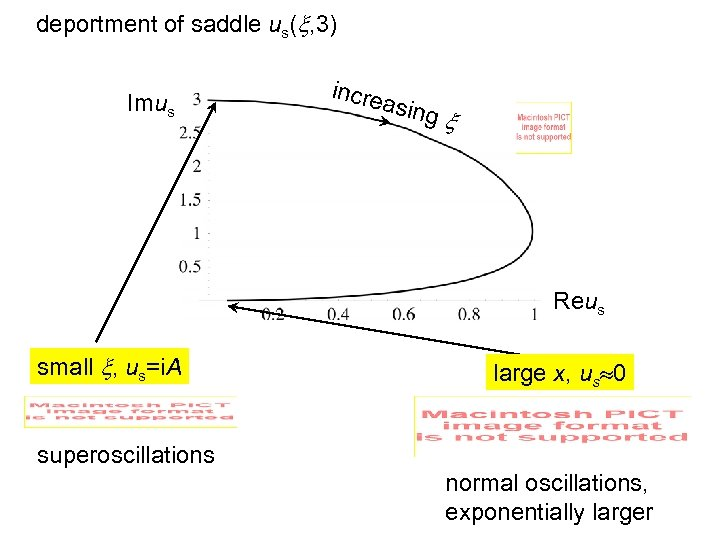deportment of saddle us( , 3) Imus incre asing Reus small , us=i. A large x, us 0 superoscillations normal oscillations, exponentially larger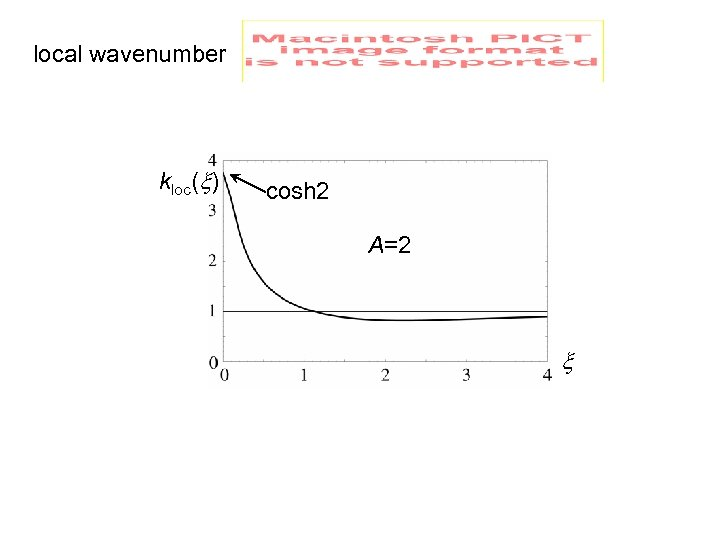local wavenumber kloc( ) cosh 2 A=2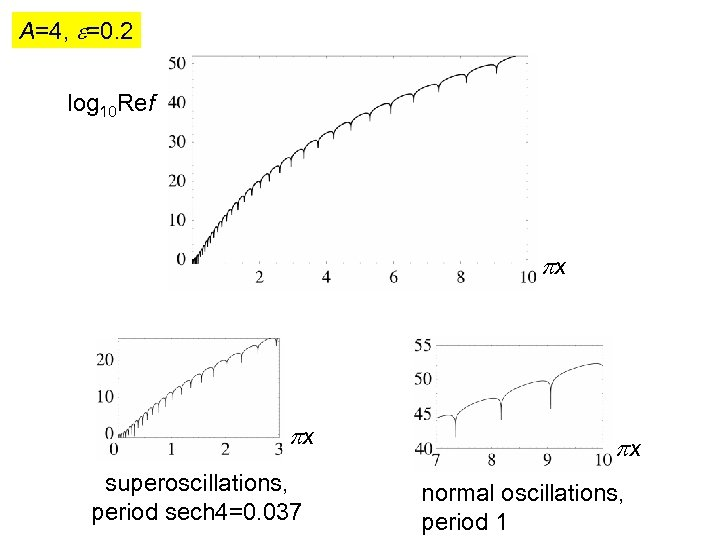A=4, =0. 2 log 10 Ref x x superoscillations, period sech 4=0. 037 x normal oscillations, period 1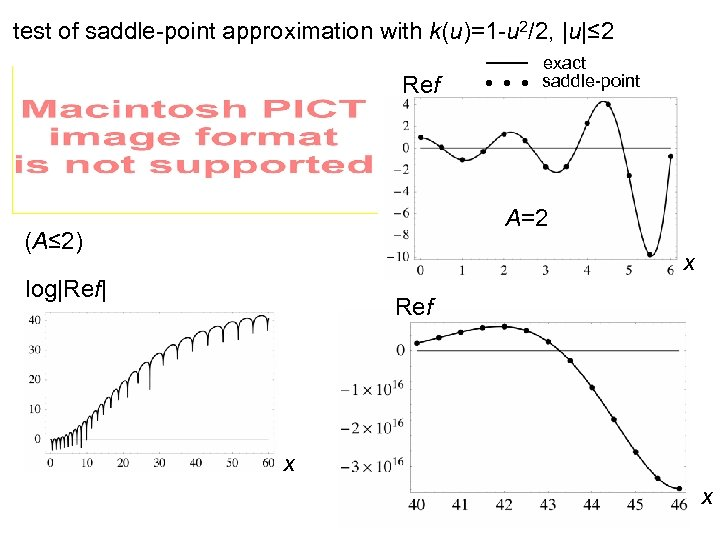test of saddle-point approximation with k(u)=1 -u 2/2, |u|≤ 2 Ref exact saddle-point A=2 (A≤ 2) x log|Ref| Ref x x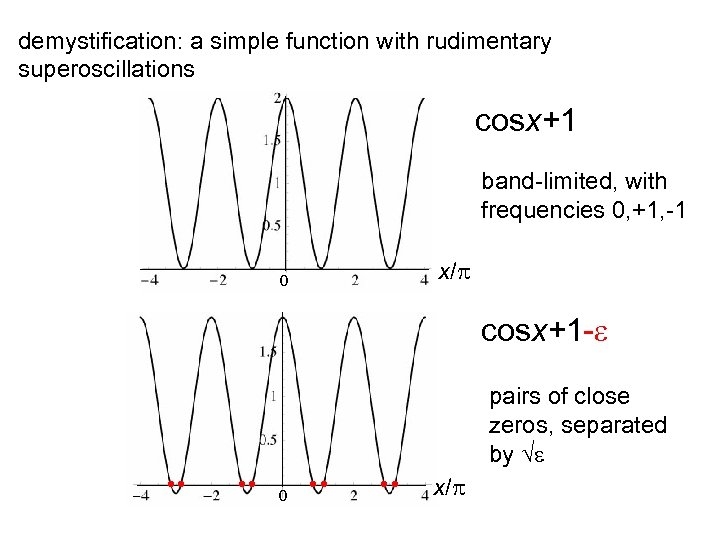demystification: a simple function with rudimentary superoscillations cosx+1 band-limited, with frequencies 0, +1, -1 0 x/ cosx+1 - pairs of close zeros, separated by 0 x/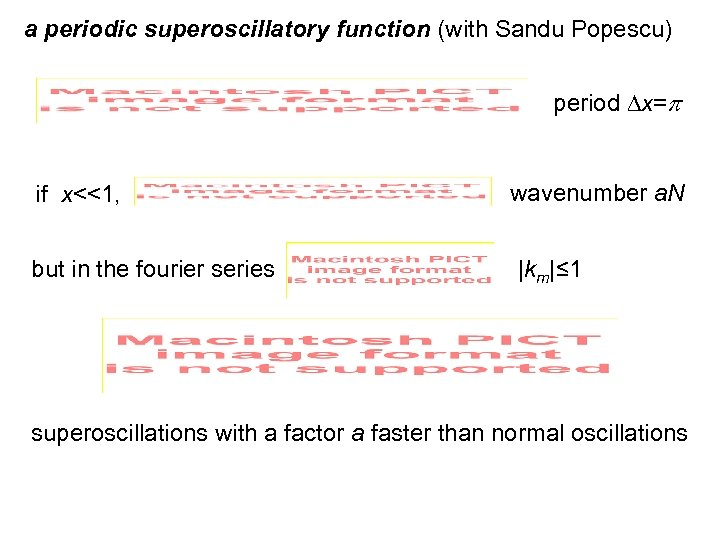a periodic superoscillatory function (with Sandu Popescu) period x= if x<<1, but in the fourier series wavenumber a. N |km|≤ 1 superoscillations with a factor a faster than normal oscillations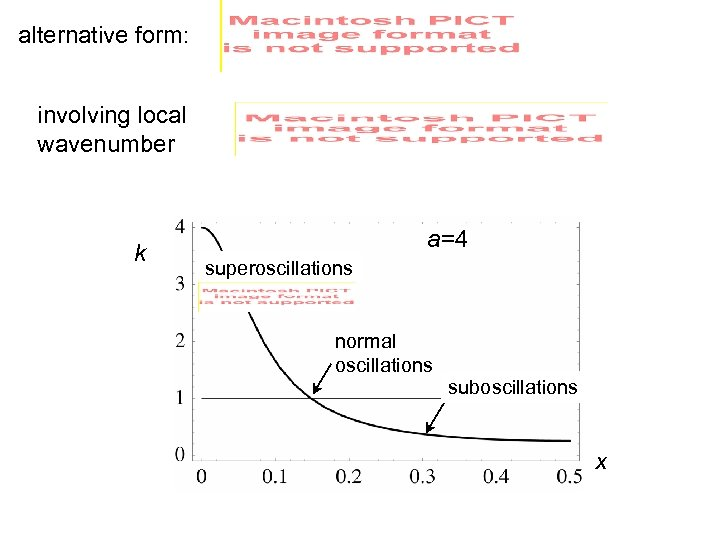alternative form: involving local wavenumber k a=4 superoscillations normal oscillations suboscillations x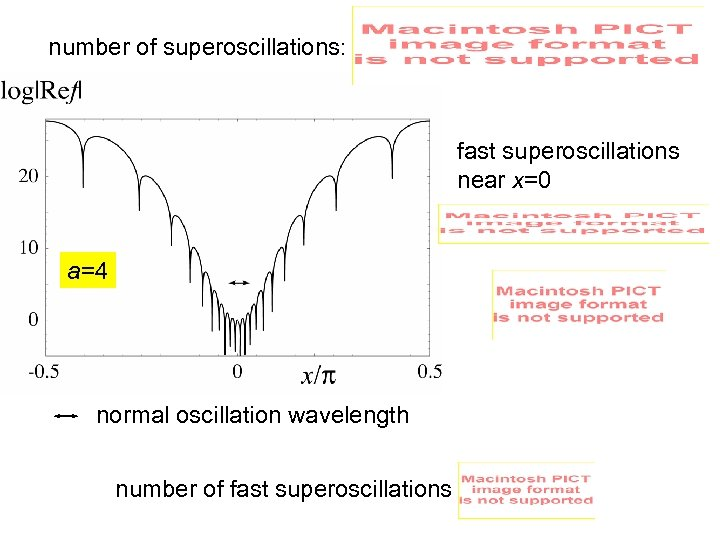number of superoscillations: fast superoscillations near x=0 a=4 normal oscillation wavelength number of fast superoscillations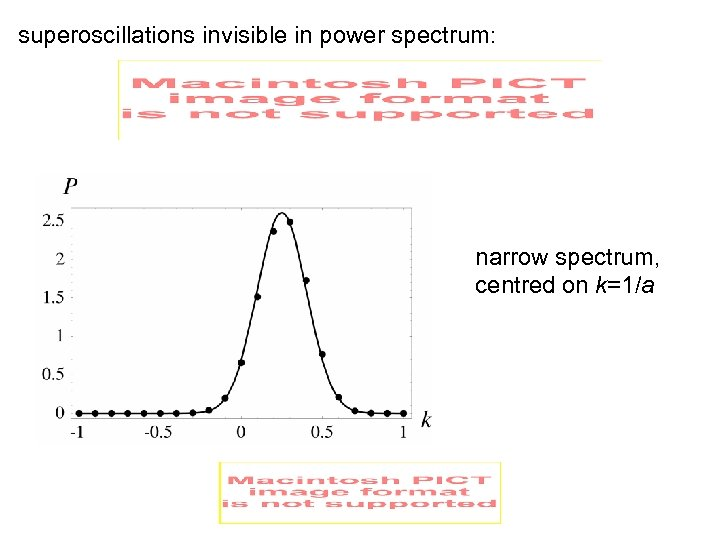superoscillations invisible in power spectrum: narrow spectrum, centred on k=1/a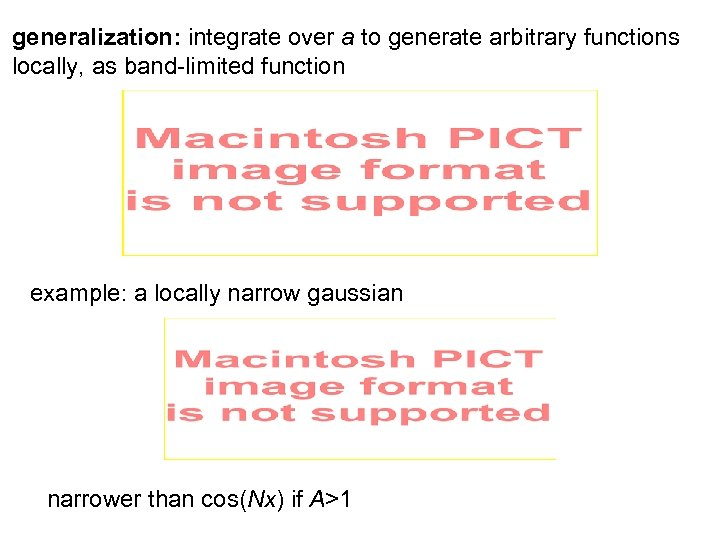generalization: integrate over a to generate arbitrary functions locally, as band-limited function example: a locally narrow gaussian narrower than cos(Nx) if A>1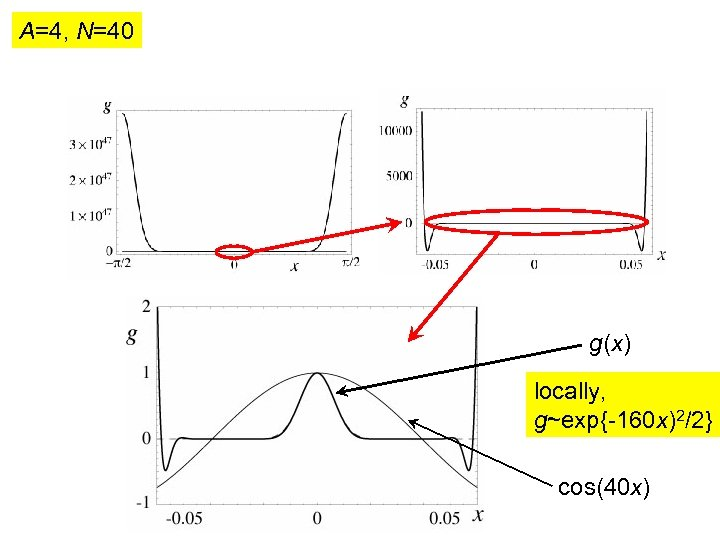A=4, N=40 g(x) locally, g~exp{-160 x)2/2} cos(40 x)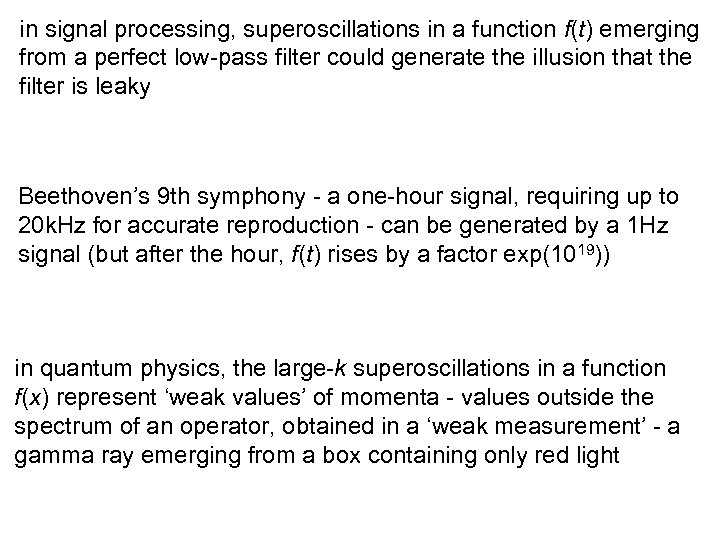in signal processing, superoscillations in a function f(t) emerging from a perfect low-pass filter could generate the illusion that the filter is leaky Beethoven’s 9 th symphony - a one-hour signal, requiring up to 20 k. Hz for accurate reproduction - can be generated by a 1 Hz signal (but after the hour, f(t) rises by a factor exp(1019)) in quantum physics, the large-k superoscillations in a function f(x) represent ‘weak values’ of momenta - values outside the spectrum of an operator, obtained in a ‘weak measurement’ - a gamma ray emerging from a box containing only red light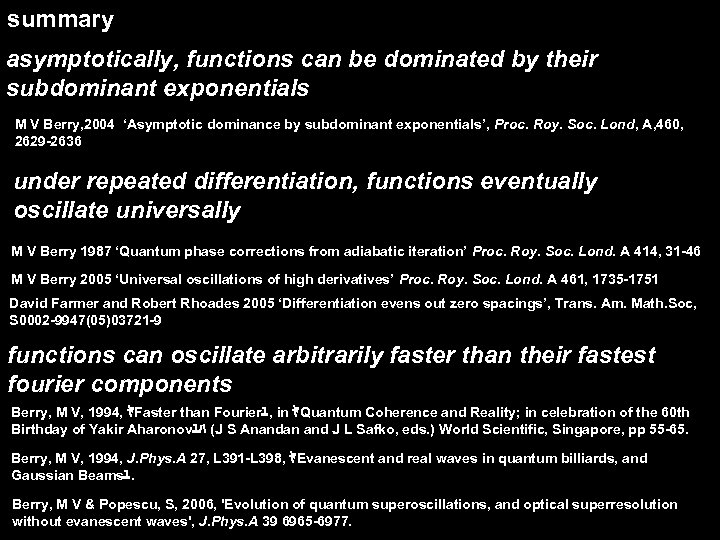summary asymptotically, functions can be dominated by their subdominant exponentials M V Berry, 2004 ‘Asymptotic dominance by subdominant exponentials’, Proc. Roy. Soc. Lond, A, 460, 2629 -2636 under repeated differentiation, functions eventually oscillate universally M V Berry 1987 ‘Quantum phase corrections from adiabatic iteration’ Proc. Roy. Soc. Lond. A 414, 31 -46 M V Berry 2005 ‘Universal oscillations of high derivatives’ Proc. Roy. Soc. Lond. A 461, 1735 -1751 David Farmer and Robert Rhoades 2005 ‘Differentiation evens out zero spacings’, Trans. Am. Math. Soc, S 0002 -9947(05)03721 -9 functions can oscillate arbitrarily faster than their fastest fourier components Berry, M V, 1994, ﾔFaster than Fourierﾕ, in ﾔQuantum Coherence and Reality; in celebration of the 60 th Birthday of Yakir Aharonovﾕﾊ (J S Anandan and J L Safko, eds. ) World Scientific, Singapore, pp 55 -65. Berry, M V, 1994, J. Phys. A 27, L 391 -L 398, ﾔEvanescent and real waves in quantum billiards, and Gaussian Beamsﾕ. Berry, M V & Popescu, S, 2006, 'Evolution of quantum superoscillations, and optical superresolution without evanescent waves', J. Phys. A 39 6965 -6977.